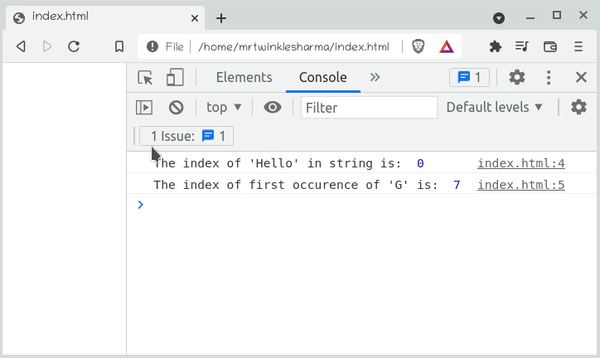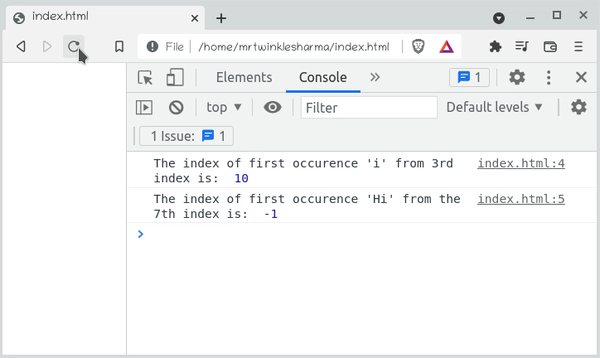# Which method returns the index within calling String object of first occurrence of specified value ?

• Last Updated : 31 Dec, 2021

In this article, we will know how to return the index of the first occurrence of the specified value(can be a string or character) in the string, also will understand their implementation through the examples.

JavaScript indexOf() Method: The indexOf() method is a built-in & case-sensitive method that returns the index of the first occurrence of the specified value in the calling string object. It will return -1 if no such value is found.

The main usage of this method is to find the existence of the particular string or character in the string because the method returns -1 if the provided search value is not present. This method can also be used to calculate the frequency of specific search value in the string.

Syntax:

`indexOf(searchValue, index)`

Parameters:

• searchValue: It is the string value to search for in the base string.
• index: It is the starting index to start the search from. By default, the index is considered as 0.

Return type: It returns the valid index of the string(which is of integer type) from where the searchValue found for the first time. Otherwise returns  -1 if the search value is not found.

Example 1: This example illustrates the search value that can be a string or any character.

## HTML

 ```<``html``>`` ` `<``head``>``    ``<``meta` `charset``=``"utf-8"` `/>``    ``<``meta` `name``=``"viewport"` `content``=``        ``"width=device-width, initial-scale=1"` `/>``    ``<``title``>``        ``Finding the index of the first``        ``occurrence of the argument string``        ``in the given string.``    `````` ` `<``body``>``    ``<``h1``>GeekforGeeks``    ``<``h3``>Javascript indexOf() Method``    ``<``script``>``        ``let myString = "Hello, GeeksforGeeks Learner!";``        ``console.log("The index of 'Hello' in string is: ",``            ``myString.indexOf("Hello"));``        ``console.log("The index of first occurence of 'G' is: ",``            ``myString.indexOf('G'));``    `````` ` ``

Explanation: In the first line, we have declared a string with initialization, and later we are calling the indexOf() method with this string as a calling object by providing the “Hello” as an argument. Now, this method will return the first occurrence of “Hello” in the string which is 0. After then, we have searched for the character ‘G’ in the string. Here, this method will return 7 because that is the first occurrence.

Output:Example 2: This example describes finding the first occurrence of the argument character or string wrt to the specific position.

## HTML

 ```<``html``>`` ` `<``head``>``    ``<``meta` `charset``=``"utf-8"` `/>``    ``<``meta` `name``=``"viewport"` `content``=``        ``"width=device-width, initial-scale=1"` `/>``    ``<``title``>``        ``Returning the index of the first``        ``occurrence of the argument string``        ``wrt the specific position.``    `````` ` `<``body``>``    ``<``h1``>GeekforGeeks``    ``<``h3``>Javascript indexOf() Method``    ``<``script``>``        ``let myString = "Hi Javascript Developer";``        ``console.log(``            ``"The index of first occurence 'i' from 3rd index is: ",``            ``myString.indexOf("i", 3));``        ``console.log(``            ``"The index of first occurence 'Hi' from the 7th index is: ",``            ``myString.indexOf('Hi', 7));``    `````` ` ``

Explanation:  Here, we are calling the indexOf() method by providing the start index of searching explicitly, In the first one, we are calling the method by providing ‘i’ and 3. The ‘i’ will be found on the 10th index if the starting search index is 3. Similarly in the second one, we have provided the ‘Hi’ for searching from the 7th index but there is no occurrence of “Hi” after the 7th index hence -1 will be returned.

Output:My Personal Notes arrow_drop_up